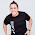(17) Pixel explosion

How does one load images into processing? Very simple. Consider this simple program that loads one of my own images from the web:
```void setup()
{
size(1280, 800);
frameRate(60);
background(0);
PImage img;
imageMode(CENTER);
image(img, width/2, height/2);
}
```

Nice, eh? But what if we wanted to create a cool effect to "explode" the image when we press the mouse button, like this:

Well, it is actually not too hard. Lets add an object that represent just one pixel. That pixel object can move and will have an initial position and color that is the color of that pixel in the image. Using the "get()" and "set()" routines in processing, we can look at what color a pixel have (get) and we can color a pixel with set. Try now to copy this program and run it. Play with the "speed" and "accel" variables. Have fun!

```ArrayList p;
int move = 0;
float speed = 0.2;
float accel = 1.01;

void setup()
{
int x, y;
color a;

size(1280, 800);
frameRate(60);
background(0);
PImage img;
imageMode(CENTER);
image(img, width/2, height/2);
stroke(200);
textSize(150);
textAlign(CENTER, CENTER);
text("Click to break", width/2, height/2);
a=color(0);
p = new ArrayList();
for (x=0; x<width; x=x+1) {
for (y=0; y<height; y=y+1) {
if (get(x, y)!=a) {
p.add(new pxl (x, y, random(-speed, speed), random(-speed, speed), get(x, y)));
}
}
}
}

void draw() {
int i;
pxl a;

background(0);
for (i=0; i<p.size(); i=i+1) {
a = (pxl) p.get(i);
a.update(move);
}
}

class pxl {
float xspeed, yspeed;
float x, y;
color p;

pxl (int a, int b, float c, float d, color e) {
x = float(a);
y = float(b);
xspeed = c;
yspeed = d;
p = e;
}

void update(int move) {
if (move==1) {
xspeed=xspeed*accel;
yspeed=yspeed*accel;
x = x + xspeed;
y = y + yspeed;
}
set(int(x), int(y), p);
}
}

void mousePressed() {
if (mouseButton == LEFT) {
move = 1;
}
}

```

1.Hello,

Would it be possible for the pixels to then recreate another image? like after the explosion, they reorganize to create another image?

Best,
Basile

1.That is possible. Maybe I'll try and post it...

2.ok great! Will be checking your page from time to time then!
thx
Basile

2.Hello,
this project seems great!
I'm trying to improve it and use a video instead of an image. Like a video explosion. But i have some problem. Would you help me?

angelica.marchesin@mail.com!

Thanks
Angelica